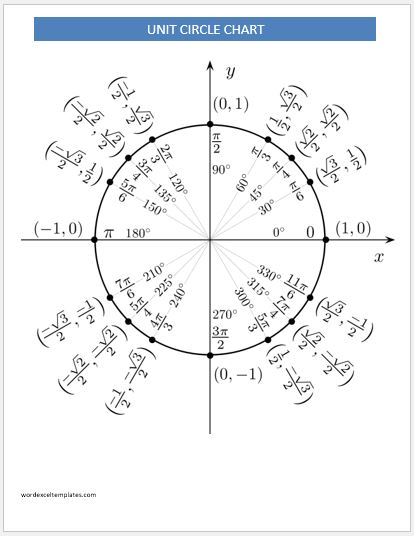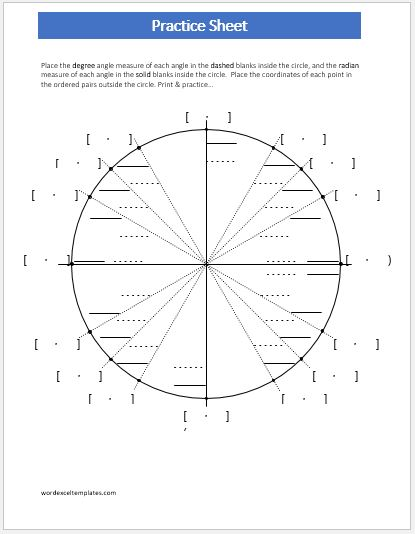# Unit Circle

This is a circle with a radius of one. It is used in mathematics and especially in the branch of trigonometry. This is basically the unit circle with the radius centered at the origin (0,0) in the coordinate system. This unit circle makes learning and understanding lengths and angles a lot easier.

## Different uses of this unit circle chart

The unit circle works on the theory of relating the angles of a triangle to the lengths of the sides of it. When a student is learning about this unit circle for the first time, this chart can help a lot in proper understanding and learning of the concept. These charts are used for different purposes, basically, this allows the user, a student or a teacher, to not just learn but understand the trigonometric functions of special angles like 30, 45, 60, and 90 degrees, and the corresponding angles. Apart from that, this chart plays a vital role in modeling periodic phenomena among other applications as well.

## Template

The template of unit circle chart can help the student in a lot of ways. This chart enables the student to draft a unit circle chart rapidly apart from other benefits that it holds. This unit circle chart can easily be drafted on a sheet of paper or on a graph paper. A unit circle chart involves a circle with two lines dividing it into quarters. Each head of the line will be labeled as 0, π/2,  π, 3π/2 and 2π starting from the right head and moving anticlockwise towards the bottom head. Each quarter must bear 3 lines, every point where the line end touches the circumference of the circle should be labeled with an angle value. For instance, the first quarter should have π/6, π/4, and π/3 each. These Radians can be calculated by using simple trigonometry. The chart, however, should be made in column format; the first column should mention all the degrees starting from 0 to 360. The other columns should be dedicated to the values of Radian, cosine, sine, tangent, cotangent, secant and cosecant against each degree mentioned.

### Preview and Detail of Templates### Unit Circle Chart Template

File: Word (.doc) 2003+ and iPad